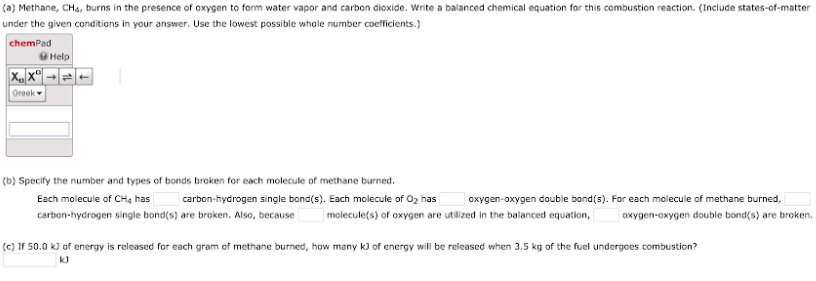# (a) Methane, CH4, burns in the presence of oxygen to form water vapor and carbon dioxide. Write a balanced equation for this combustion reaction. (Include states-of-matter under the given conditions in your answer. Use the lowest possible whole number coefficients.) (b) Specify the number and types of bonds broken for each molecule of methane burned. Each molecule of CH4 has ___ carbon-hydrogen single bond(s). Each molecule of O2 has ___ oxygen-oxygen double bond(s). For each molecule of methane burned, ___ carbon-hydrogen single bond(s) are broken. Also, because ___ molecule(s) of oxygen are utilized in the balanced equation, ___ oxygen-oxygen double bond(s) are broken. (c) If 50.0 kJ of energy is released for each gram of methane burned, how many kJ of energy will be released when 3.5 kg of the fuel undergoes combustion?